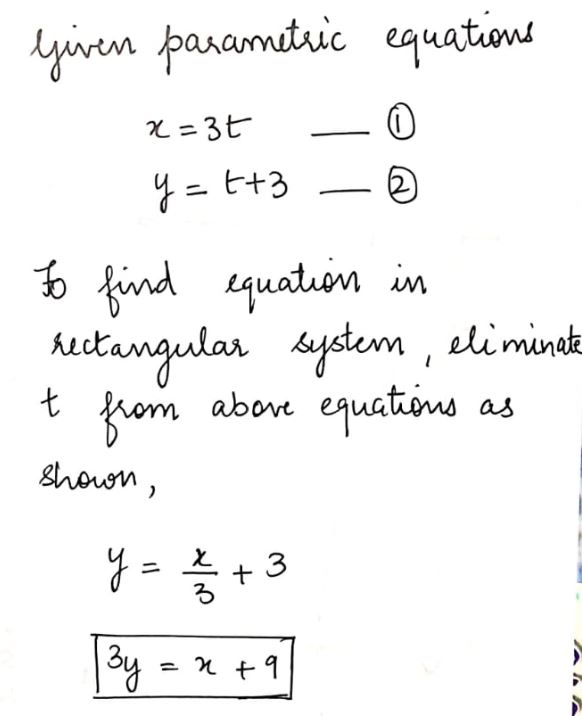# Find the rectangular equation for given parametric equationsx= 3t      y=t+3

Question
89 views

Find the rectangular equation for given parametric equations

x= 3t      y=t+3

check_circle

Step 1...

### Want to see the full answer?

See Solution

#### Want to see this answer and more?

Solutions are written by subject experts who are available 24/7. Questions are typically answered within 1 hour.*

See Solution
*Response times may vary by subject and question.
Tagged in